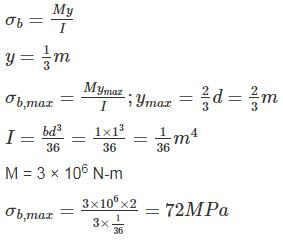Courses

# Solid Mechanics- 1

## 10 Questions MCQ Test GATE Civil Engineering (CE) 2022 Mock Test Series | Solid Mechanics- 1

Description
This mock test of Solid Mechanics- 1 for Civil Engineering (CE) helps you for every Civil Engineering (CE) entrance exam. This contains 10 Multiple Choice Questions for Civil Engineering (CE) Solid Mechanics- 1 (mcq) to study with solutions a complete question bank. The solved questions answers in this Solid Mechanics- 1 quiz give you a good mix of easy questions and tough questions. Civil Engineering (CE) students definitely take this Solid Mechanics- 1 exercise for a better result in the exam. You can find other Solid Mechanics- 1 extra questions, long questions & short questions for Civil Engineering (CE) on EduRev as well by searching above.
QUESTION: 1

### In the tension test of a bar the fractured surface shows a truncated cone, the material may be:

Solution: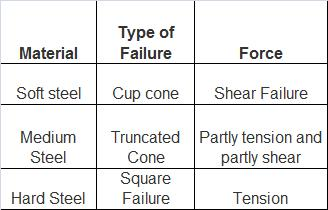QUESTION: 2

### In the engineering stress-strain curve for mild steel, the Ultimate Tensile Strength (UTS) refers to

Solution:

The engineering stress-strain curve for mild steel is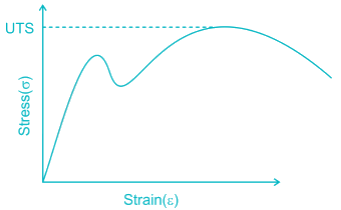Ultimate tensile strength represents the maximum stress that a material can withstand without Fracture.

QUESTION: 3

### _______ is the capacity of material to absorb energy when it is elastically deformed and then upon unloading, to have this energy recovered.

Solution:

Resilience: It is energy absorbed by a member in elastic region. It denotes the capacity of material to absorb energy when it is elastically deformed and then upon unloading, to release this energy.

Toughness: It is energy absorbed by member just before its fracture.

QUESTION: 4

The shear modulus of a material is half of its Young’s modulus. What is the value of its Poisson’s ratio?

Solution: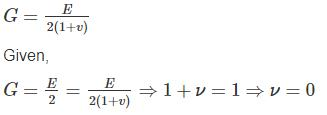QUESTION: 5

A mild steel wire is 10 mm in diameter and 1 m long. If the wire is subjected to an axial tensile load 10 kN, find an extension of the road (Take E = 200 × 10Pa) :

Solution: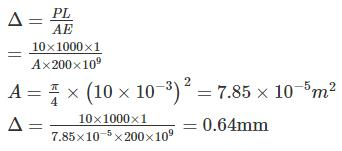QUESTION: 6

The ratio of strengths of solid to hollow shafts, both having outside diameter D and hollow having inside diameter D/2, in torsion, is

Solution: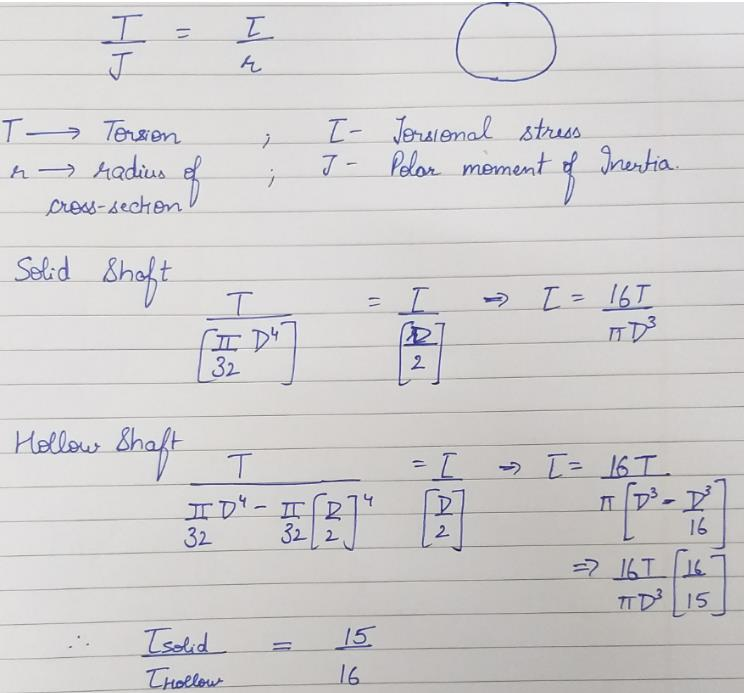QUESTION: 7

A simply supported beam is subjected to a uniformly distributed load. Which one of the following statements is true?

Solution: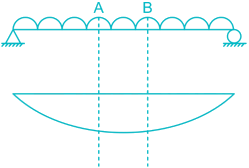For section AB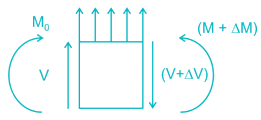For equilibrium, ∑M0 = 0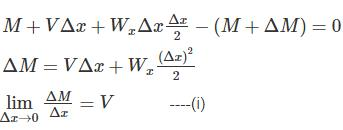For M to be maximum or minimum ⇒ dM/dx = 0

Hence dM/dx = V = 0

QUESTION: 8

A steel plate of side ‘a’ (2d) is enclosed in a closely fitting rigid support as shown. Assume friction between plate and support is zero. Determine the free expansion in x - direction of the plate due to heating by ΔT – (Poison ratio is μ & thermal co - eff. α)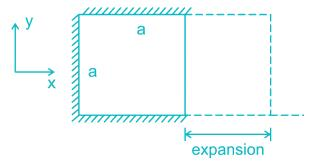Solution:

σx = 0
σy = −αTE
εy = −αT
εx = −με= μαT = δa/a
ϵx = Free expansion due to temperature change + Expansion due to lateral strain

= a α Δ T + μ a α Δ T (due to y direction)

= a α Δ T (1 + μ)

*Answer can only contain numeric values
QUESTION: 9

For a cylindrical bar of 30 mm dia& 900 mm length, during a tension test, it is found that longitudinal strain is 4 times of lateral strain. The bulk modulus for the bar is (X) × 105 N/mm2  (if E = 3 × 105 N/mm2) The value if X is ___________

Solution:

E = 3 × 105 N/mm2 & E = 3k (1 – 2μ).....(1)
From (1) we get K = E/3(1−2μ) [∴μ=0.25 given]
put μ=0.25 in equation 1,we get
k=2*105N/mm2.

QUESTION: 10

A cantilever beam has the cross-section of an isosceles triangle and is loaded as shown in figure. If the moment of inertia of the cross-section Izz = 1/36m4, then the maximum bending stress is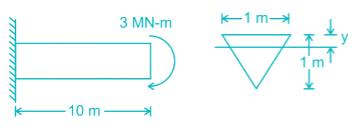Solution: